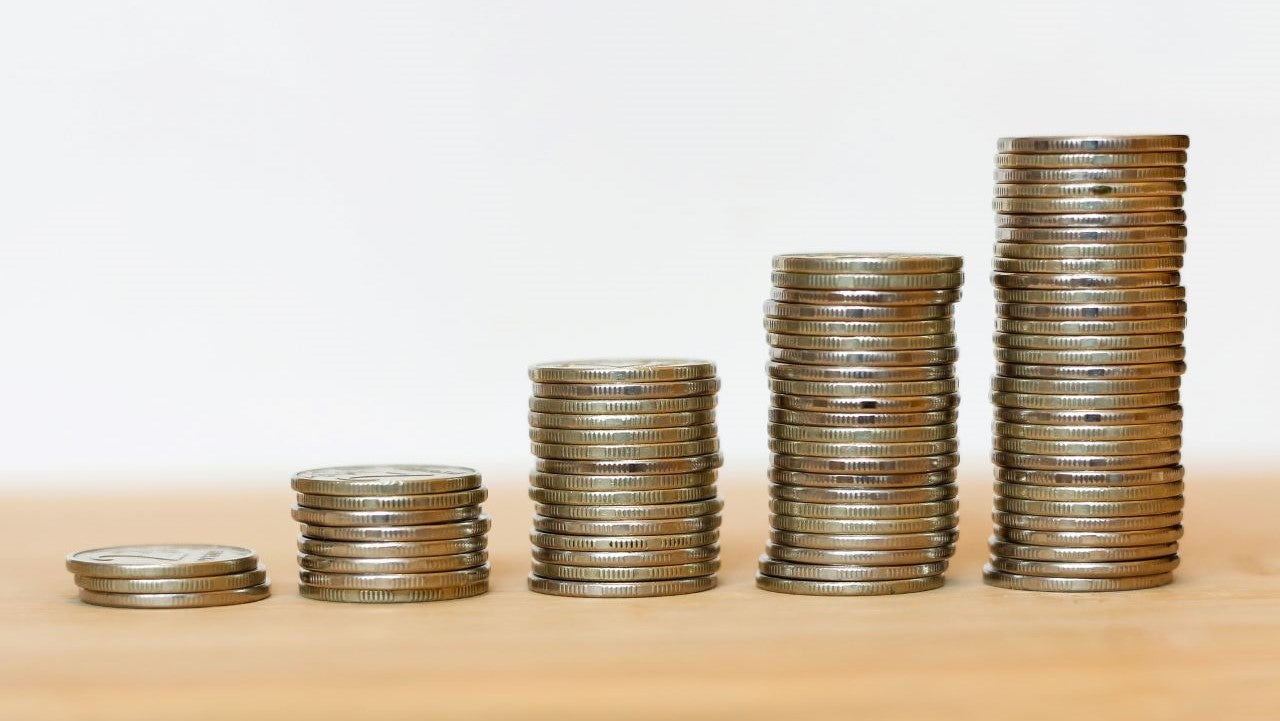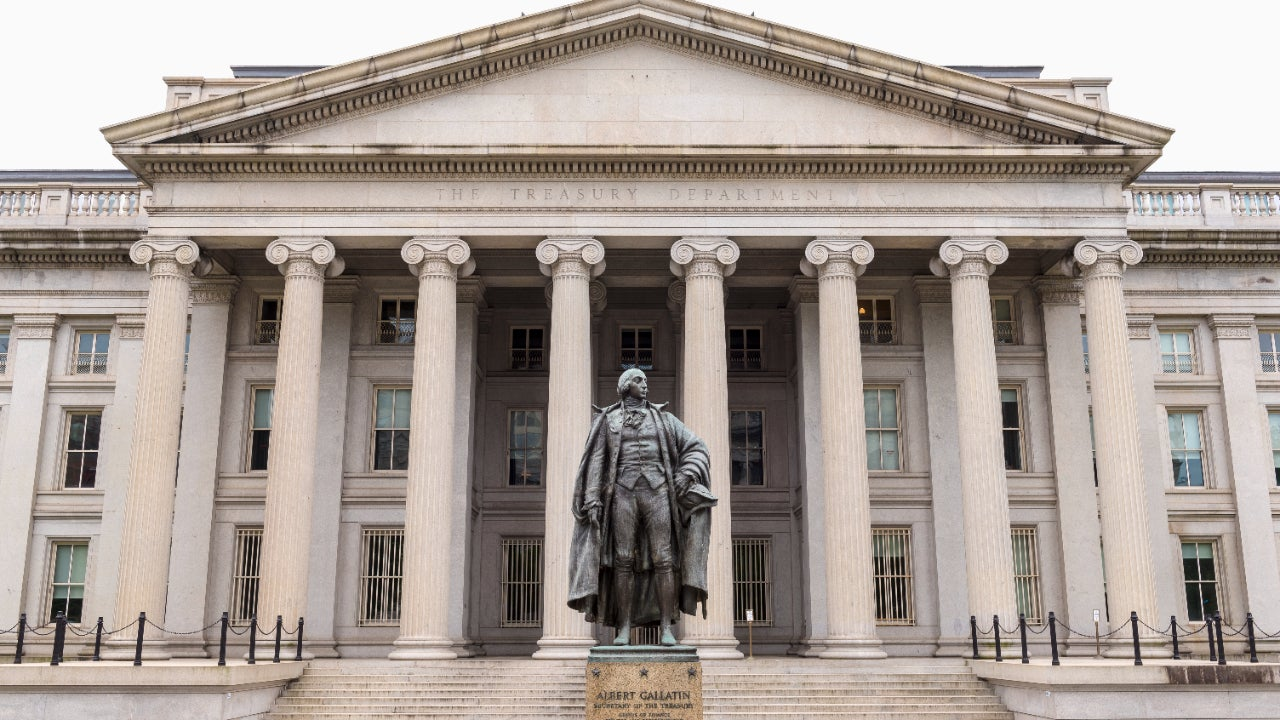# Rule of 72: What it is and how to use it## Why you can trust Bankrate

While we adhere to strict , this post may contain references to products from our partners. Here's an explanation for .

## What is the Rule of 72?

The Rule of 72 is a calculation that estimates the number of years it takes to double your money at a specified rate of return. If, for example, your account earns 4 percent, divide 72 by 4 to get the number of years it will take for your money to double. In this case, 18 years.

The same calculation can also be useful for inflation, but it will reflect the number of years until the initial value has been cut in half, rather than doubling.

The Rule of 72 is derived from a more complex calculation and is an approximation, and therefore it isn’t perfectly accurate. The most accurate results from the Rule of 72 are based at the 8 percent interest rate, and the farther from 8 percent you go in either direction, the less precise the results will be. Still, this handy formula can help you get a better grasp on how much your money may grow, assuming a specific rate of return.

## The formula for the Rule of 72

The Rule of 72 can be expressed simply as:

Years to double = 72 / rate of return on investment (or interest rate)

There are a few important caveats to understand with this formula:

• The interest rate shouldn’t be expressed as a decimal out of 1, such as 0.07 for 7 percent. It should just be the number 7. So, for example, 72/7 is 10.3, or 10.3 years.
• The Rule of 72 is focused on compounding interest that compounds annually.
• For simple interest, you’d simply divide 1 by the interest rate expressed as a decimal. If you had \$100 with a 10 percent simple interest rate with no compounding, you’d divide 1 by 0.1, yielding a doubling rate of 10 years.
• For continuous compounding interest, you’ll get more accurate results by using 69.3 instead of 72. The Rule of 72 is an estimate, and 69.3 is harder for mental math than 72, which divides easily by 2, 3, 4, 6, 8, 9, and 12. If you have a calculator, however, use 69.3 for slightly more accurate results.
• The farther you diverge from an 8 percent return, the less accurate your results will be. The Rule of 72 works best in the range of 5 to 12 percent, but it’s still an approximation.
• To calculate based on a lower interest rate, like 2 percent, drop the 72 to 71; to calculate based on a higher interest rate, add one to 72 for every three percentage point increase. So, for example, use 74 if you’re calculating doubling time for 18 percent interest.

## How the Rule of 72 works

The actual mathematical formula is complex and derives the number of years until doubling based on the time value of money.

You’d start with the future value calculation for periodic compounding rates of return, a calculation that helps anyone interested in calculating exponential growth or decay:

FV = PV*(1+r)t

FV is future value, PV is present value, r is the rate and the t is the time period. To isolate t when it’s located in an exponent, you can take the natural logarithms of both sides. Natural logarithms are a mathematical way to solve for an exponent. A natural logarithm of a number is the number’s own logarithm to the power of e, an irrational mathematical constant that is approximately 2.718. With the example of a doubling of \$10, deriving the Rule of 72 would look like this:

20 = 10*(1+r)t

20/10 = 10*(1+r)t/10

2 = (1+r)t

ln(2) = ln((1+r)t)

ln(2) = r*t

The natural log of 2 is 0.693147, so when you solve for t using those natural logarithms, you get t = 0.693147/r.

The actual results aren’t round numbers and are closer to 69.3, but 72 easily divides for many of the common rates of return that people get on their investments, so 72 has gained popularity as a value to estimate doubling time.

For more precise data on how your investments are likely to grow, use a compound interest calculator that’s based on the full formula.

## How to use the Rule of 72 for your investment planning

Most families aim to continue investing over time, often monthly. You can project how long it takes to get to a given target amount if you have an average rate of return and a current balance. If, for example, you have \$100,000 invested today at 10 percent interest, and you are 22 years away from retirement, you can expect your money to double approximately three times, going from \$100,000 to \$200,000, then to \$400,000, and then to \$800,000.

If your interest rate changes or you need more money because of inflation or other factors, use the results from the Rule of 72 to help you decide how to keep investing over time.

You can also use the Rule of 72 to make choices about risk versus reward. If, for example, you have a low-risk investment that yields 2 percent interest, you can compare the doubling rate of 36 years to that of a high-risk investment that yields 10 percent and doubles in seven years.

Many young adults who are starting out choose high-risk investments because they have the opportunity to take advantage of high rates of return for multiple doubling cycles. Those nearing retirement, however, will likely opt to invest in lower-risk accounts as they near their target amount for retirement because doubling is less important than investing in more secure investments.

## Rule of 72 during inflation

Investors can use the rule of 72 to see how many years it will take to cut in half their purchasing power due to inflation. For example, if inflation is around 8 percent (as during the middle of 2022), you can divide 72 by the rate of inflation to get 9 years until the purchasing power of your money is reduced by 50 percent.

72/8 = 9 years to lose half your purchasing power.

The Rule of 72 allows investors to realize the severity of inflation concretely. Inflation might not remain elevated for such a long period of time, but it has done so in the past over a multi-year period, really hurting the purchasing power of accumulated assets.

## Bottom Line

The Rule of 72 is an important guideline to keep in mind when considering how much to invest. Investing even a small amount can make a big impact if you start early, and the effect can only increase the more you invest, as the power of compounding works its magic. You can also use the Rule of 72 to assess how quickly you can lose purchasing power during periods of inflation.

NoteBankrate’s Georgina Tzanetos contributed to a recent update of this story.

Laura Leavitt is a former contributor to Bankrate.
Edited by
Senior investing and wealth management reporter
Reviewed by
Senior wealth manager, LourdMurray

• ## Best compound interest investments• ## 6 investment formulas for financial success• ## Treasury Inflation-Protected Securities: What are TIPS?